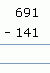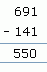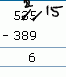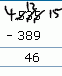Email us to get an instant 20% discount on highly effective K-12 Math & English kwizNET Programs!

#### Online Quiz (WorksheetABCD)

Questions Per Quiz = 2 4 6 8 10

### MEAP Preparation - Grade 3 Mathematics2.10 Subtracting - Three Digits (WIZ Math)

Subtraction without borrow
Method:
1. Start from the units place
2. Subtract the numbers in the units place
3. Subtract the numbers in the tens place
4. Subtract the numbers in the hundreds place
 Question Step 1 Step 2 Step 3Subtraction with borrow
Sometimes when you subtract you need to regroup 1 ten as 10 ones.
 Question Step 1 Step 2 Step 3• Start from the ones place
• In the ones place, since we cannot subtract 9 from 5, we borrow 1 from 3 and make it 2.
• In ones place we get 15-9 = 6
• In the tens place, since we have taken out 1 from 3, we have 3-1=2
• Since we cannot subtract 8 from 2, we borrow 1 from 5 and make it 4.
• In hundreds place, we have 5-3=1

Directions: Answer the following questions. Also write at least ten examples of your own.
 Question 1: 831 - 168

 Question 2: 257 - 93

 Question 3: 733 - 87

 Question 4: 583 - 181

 Question 5: 504 - 325

Question 6: This question is available to subscribers only!

Question 7: This question is available to subscribers only!

Question 8: This question is available to subscribers only!

Question 9: This question is available to subscribers only!

Question 10: This question is available to subscribers only!

#### Subscription to kwizNET Learning System offers the following benefits:

• Unrestricted access to grade appropriate lessons, quizzes, & printable worksheets
• Instant scoring of online quizzes
• Progress tracking and award certificates to keep your student motivated
• Unlimited practice with auto-generated 'WIZ MATH' quizzes